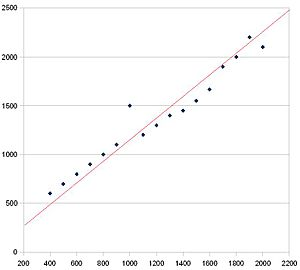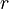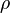# Correlation facts for kids

Kids Encyclopedia Facts

In statistics and probability theory, correlation is a way to indicate how closely related two sets of data are.

Correlation does not always mean that one causes the other. In fact, it is very possible that there is a third factor involved.

Correlation usually has one of two directions. These are positive or negative. If it is positive, then the two sets go up together. If it is negative, then one goes up while the other goes down.

Lots of different measurements of correlation are used for different situations. For example, on a scatter graph, people draw a line of best fit to show the direction of the correlation.This scatter graph has positive correlation. You can tell because the trend is up and right. The red line is a line of best fit.

## Explaining correlation

Strong and weak are words used to describe the strength of correlation. If there is strong correlation, then the points are all close together. If there is weak correlation, then the points are all spread apart. There are ways of making numbers show how strong the correlation is. These measurements are called correlation coefficients. The best known is the Pearson product-moment correlation coefficient, sometimes denoted by$r$ or its Greek equivalent$\rho$. You put in data into a formula, and it gives you a number between -1 and 1. If the number is 1 or −1, then there is strong correlation. If the answer is 0, then there is no correlation. Another kind of correlation coefficient is Spearman's rank correlation coefficient.

## Correlation vs causation

Correlation does not always mean that one thing causes the other (causation), because there might be something else that is at play.

For example, on hot days people buy ice cream, and people also go to the beach where some are eaten by sharks. There is a correlation between ice cream sales and shark attacks (they both go up as the temperature goes up in this case). But just because ice cream sales go up does not mean ice cream sales cause (causation) more shark attacks or vice versa.

Because correlation does not imply causation, scientists, economists, etc. will test their theories by creating isolated environments where only one factor is changed (where this is possible). However, politicians, salesmen, news outlets and others often suggest that a particular correlation implies causation. This may be due to ignorance or a wish to persuade. Thus, a news report may attract attention by saying that people who consume a particular product more often have a particular health problem, implying a causation that could be actually due to something else.

## Related pages

• Cohen, J., Cohen P., West, S.G., & Aiken, L.S. (2003). Applied multiple regression/correlation analysis for the behavioral sciences. (3rd ed.) Hillsdale, NJ: Lawrence Erlbaum Associates.

## Images for kidsIn Spanish: Correlación para niños